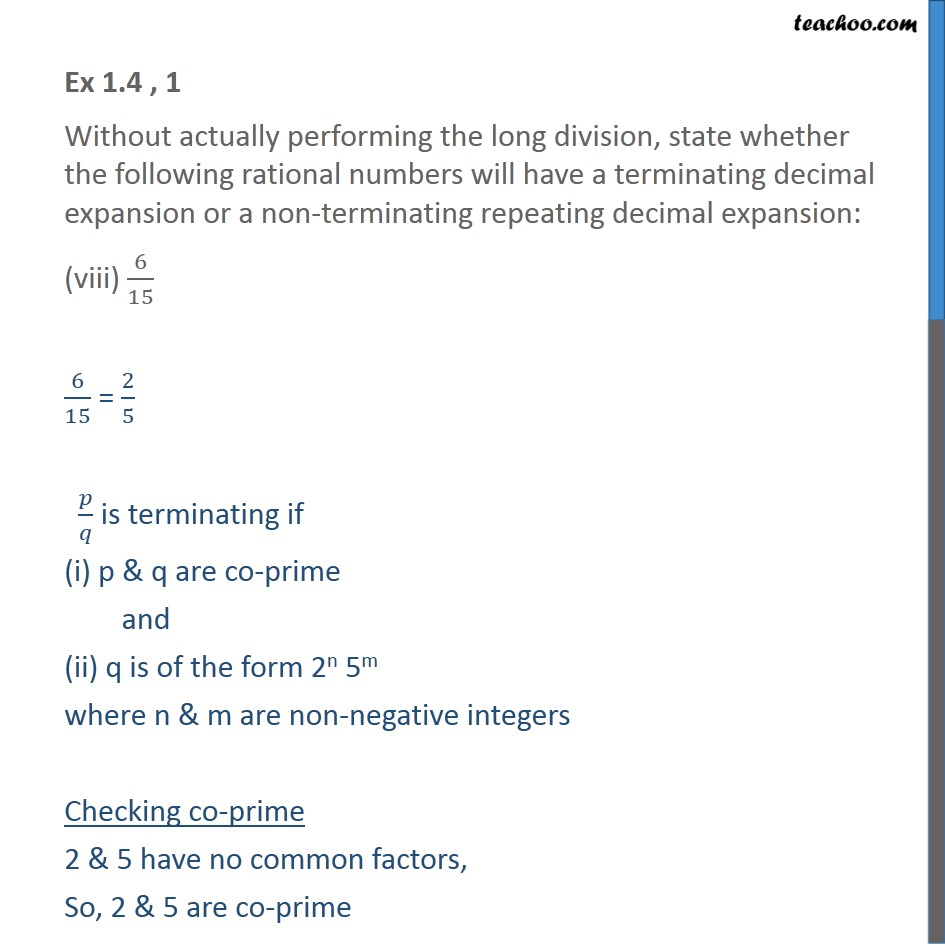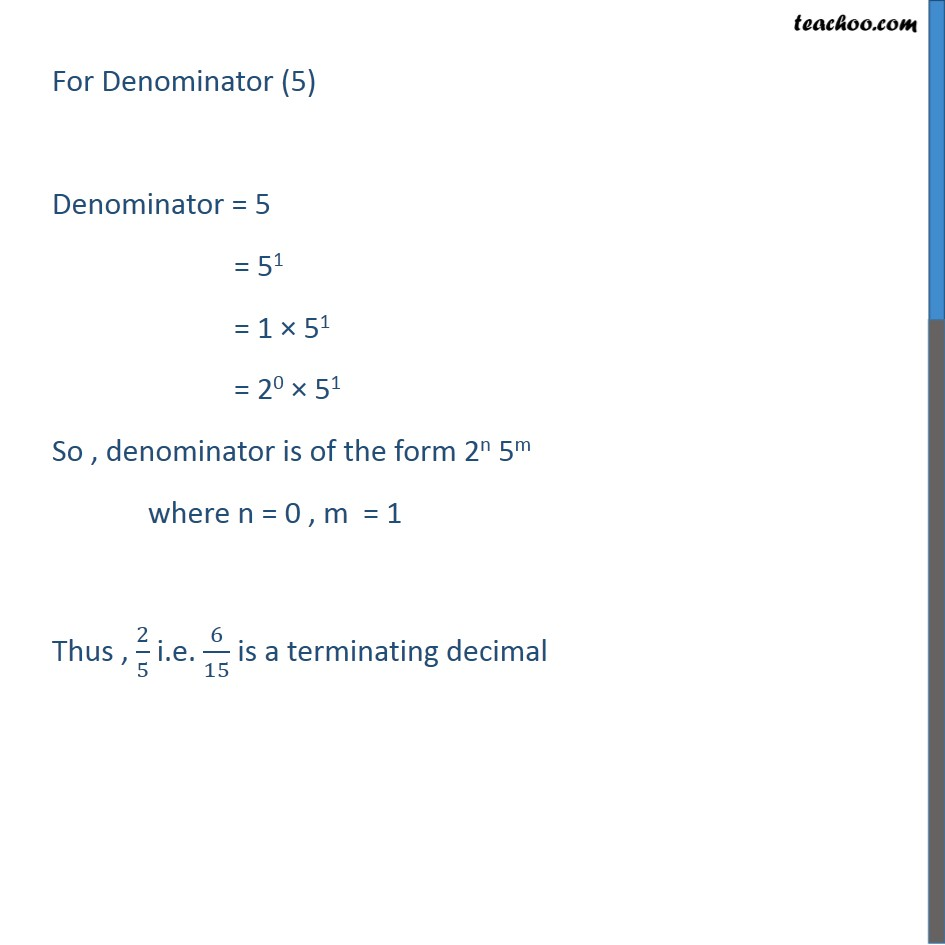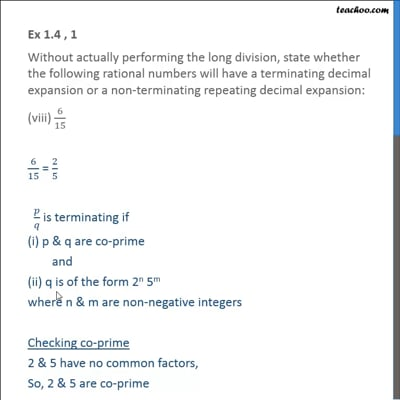Ex 1.4

Chapter 1 Class 10 Real Numbers
Serial order wiseThis video is only available for Teachoo black users

Introducing your new favourite teacher - Teachoo Black, at only ₹83 per month

### Transcript

Ex 1.4 , 1 Without actually performing the long division, state whether the following rational numbers will have a terminating decimal expansion or a non-terminating repeating decimal expansion: (viii) 6/15 6/15 = 2/5 𝑝/𝑞 is terminating if (i) p & q are co-prime and (ii) q is of the form 2n 5m where n & m are non-negative integers Checking co-prime 2 & 5 have no common factors, So, 2 & 5 are co-prime For Denominator (5) Denominator = 5 = 51 = 1 × 51 = 20 × 51 So , denominator is of the form 2n 5m where n = 0 , m = 1 Thus , 2/5 i.e. 6/15 is a terminating decimal